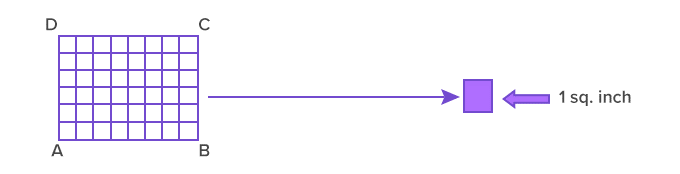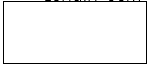# AREA OF RECTAMGLE AND SQUARE

##### HELLO, WELCOME BACK TO CLASS

A rectangle is a shape/polygon with four, four vertices, and four. The two opposite sides and parallel to each other. Some examples of rectangular figures are agricultural fields, parks, tiles, daily life objects such as pans, glass, table, serving tray, etc. The area of a polygon is the number of square units inside the polygon. To understand the difference between perimeter and area, think of perimeter as the length of fence needed to enclose the yard, whereas area is the space inside the yard. Perimeter is 1-dimensional and is measured in linear units such as inches, feet or meters. Area is 2-dimensional: it has a length and a width. Area is measured in square units such as square inches, square feet or square meters.To find the area of a rectangle, multiply the length by the width. The formula is:

A = L * W where A is the area, L is the length, W is the width, and * means multiply.

To derive the area of a rectangle, we use the unit squares. Divide the rectangle ABCD into unit squares, as shown. The area of a rectangle ABCD is the total number of unit squares contained within it.Rectangle ABCD in unit squares with each of 1 sq. inch

Thus, the total area of the rectangle ABCD is 48 sq. inch.

Perimeter of Rectangle

Find the perimeter and area

Length=5cm              L+L+B+B or 2(L+B)         5cm7CM

Perimeter: 2(L+B) or L+L+B+B

(5+5+7+7)cm or 2(5+7)cm

24cm  or             2(12)cm

24cm

Area=L×B

5×7=

35cm2

Square

L+L+L+L or 4×L=4L5cm                                           Length=5cm                5+5+5+5 or 4×5

5cm

Perimeter=4×L or L+L+L+L

4×5=        5+5+5+5                                       20cm   or     20cm

area=L×L

5×5=

25cm

Area of Square

square is a rectangle with 4 equal sides. To find the area of a square, multiply the length of one side by itself.

Area of a square is defined as the number of square units needed to fill a square. In general, the area is defined as the region occupied inside the boundary of a flat object or figure. The measurement is done in square units with the standard unit being square meters (m2). For the computation of area, there are pre-defined formulas for squares, rectangles, circle, triangles, etc. In this article, you will learn about the area of a square.

What is Area?

Area is the space covered by the object. It is the region occupied by any shape. While measuring the area of a square, we consider only the length of its side. All the sides of a square are equal and hence, its area is equal to the square of side. Similarly, we can find the area of the other shapes such as rectangle, parallelogram, triangle or any polygon, based on its sides. Area of the surface is calculated based on the radius or the distance of its outer line from the axis for

Thus, the area of the square is 25 square cm, which can be written as 5 cm × 5 cm, that is, side ×  side.

From the above discussion, it can be inferred that the formula can give the area of a square is:

Area of a Square = Side ×  Side

Therefore, the area of square = Side2  square units

and the perimeter of a square = 4 ×  side units

Here some of the unit conversion lists are provided for reference. Some conversions of units:

• 1 m = 100 cm
• 1 sq. m = 10,000 sq. cm
• 1 km = 1000 m
• 1 sq. km = 1,000,000 sq. m

Area of a Square Sample Problems

Example 1: Find the area of a square clipboard whose side measures 120 cm.

Solution:

Side of the clipboard = 120 cm = 1.2 m

Area of the clipboard = side  × side

= 120 cm  ×120 cm

= 14400 sq. cm

= 1.44 sq. m

Example 2: The side of a square wall is 75 m. What is the cost of painting it at the rate of Rs. 3 per sq. m?

Solution:

Side of the wall = 75 m

Area of the wall = side × side = 75 m × 75 m = 5625 sq. m

For 1 sq. m, the cost of painting = Rs. 3

Thus, for 5625 sq. m, the cost of painting = Rs. 3 × 5625 = Rs 16875

Example 3: A courtyard’s floor which is 50 m long and 40 m wide is to be covered by square tiles. The side of each tile is 2 m. Find the number of tiles required to cover the floor.

Length of the floor = 50 m

The breadth of the floor = 40 m

Area of the floor = length × breadth = 50 m  ×40 m = 2000 sq. m

Side of one tile = 2 m

Area of one tile = side ×side = 2 m  × 2 m = 4 sq. m

No. of tiles required = area of floor/area of a tile = 2000/4 = 500 tiles

To learn and practise more problems related to the area of a square, download BYJU’S-The Learning App

Quiz

1. A courtyard’s floor which is 50 m long and 40 m wide is to be covered by square tiles. The side of each tile is 2 m. Find the number of tiles required to cover the floor.
2. The side of a square wall is 75 m. What is the cost of painting it at the rate of Rs. 3 per sq. m?
Your Opinion Matters! Quickly tell us how to improve your Learning Experience HERE